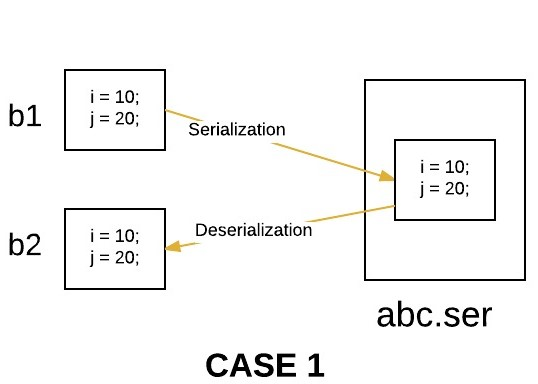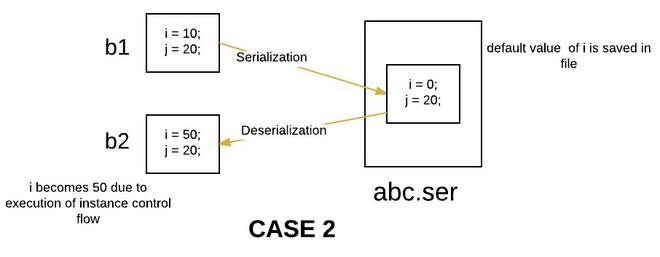Open In App

# Object Serialization with Inheritance in Java

Prerequisite: Serialization, Inheritance

Serialization is a mechanism of converting the state of an object into a byte stream. The byte array can be the class, version, and internal state of the object.

Deserialization is the reverse process where the byte stream is used to recreate the actual Java object in memory. This mechanism is used to persist the object.

There are some cases of Serialization with respect to inheritance:

#### Case 1: If the superclass is serializable, then subclass is automatically serializable

If the superclass is Serializable, then by default, every subclass is serializable. Hence, even though subclass doesn’t implement Serializable interface( and if its superclass implements Serializable), then we can serialize subclass object.

## Java

 `// Java program to demonstrate ``// that if superclass is ``// serializable then subclass ``// is automatically serializable `` ` `import` `java.io.FileInputStream; ``import` `java.io.FileOutputStream; ``import` `java.io.ObjectInputStream; ``import` `java.io.ObjectOutputStream; ``import` `java.io.Serializable; `` ` `// superclass A ``// implementing Serializable interface ``class` `A ``implements` `Serializable ``{ ``    ``int` `i; ``     ` `    ``// parameterized constructor ``    ``public` `A(``int` `i) ``    ``{ ``        ``this``.i = i; ``    ``} ``     ` `} `` ` `// subclass B ``// B class doesn't implement Serializable ``// interface. ``class` `B ``extends` `A ``{ ``    ``int` `j; ``     ` `    ``// parameterized constructor ``    ``public` `B(``int` `i, ``int` `j) ``    ``{ ``        ``super``(i); ``        ``this``.j = j; ``    ``} ``} `` ` `// Driver class ``public` `class` `Test ``{ ``    ``public` `static` `void` `main(String[] args) ``            ``throws` `Exception ``    ``{ ``        ``B b1 = ``new` `B(``10``,``20``); ``         ` `        ``System.out.println(``"i = "` `+ b1.i); ``        ``System.out.println(``"j = "` `+ b1.j); ``         ` `        ``/* Serializing B's(subclass) object */``         ` `        ``//Saving of object in a file ``        ``FileOutputStream fos = ``new` `FileOutputStream(``"abc.ser"``); ``        ``ObjectOutputStream oos = ``new` `ObjectOutputStream(fos); ``             ` `        ``// Method for serialization of B's class object ``        ``oos.writeObject(b1); ``             ` `        ``// closing streams ``        ``oos.close(); ``        ``fos.close(); ``             ` `        ``System.out.println(``"Object has been serialized"``); ``         ` `        ``/* De-Serializing B's(subclass) object */``         ` `        ``// Reading the object from a file ``        ``FileInputStream fis = ``new` `FileInputStream(``"abc.ser"``); ``        ``ObjectInputStream ois = ``new` `ObjectInputStream(fis); ``             ` `        ``// Method for de-serialization of B's class object ``        ``B b2 = (B)ois.readObject(); ``             ` `        ``// closing streams ``        ``ois.close(); ``        ``fis.close(); ``             ` `        ``System.out.println(``"Object has been deserialized"``); ``         ` `        ``System.out.println(``"i = "` `+ b2.i); ``        ``System.out.println(``"j = "` `+ b2.j); ``    ``} ``}`

Output:

```i = 10
j = 20
Object has been serialized
Object has been deserialized
i = 10
j = 20```#### Case 2: If a superclass is not serializable, then subclass can still be serialized

Even though the superclass doesn’t implement a Serializable interface, we can serialize subclass objects if the subclass itself implements a Serializable interface. So we can say that to serialize subclass objects, superclass need not be serializable. But what happens with the instances of superclass during serialization in this case. The following procedure explains this.

#### Case 2(a): What happens when a class is serializable, but its superclass is not?

Serialization: At the time of serialization, if any instance variable inherits from the non-serializable superclass, then JVM ignores the original value of that instance variable and saves the default value to the file.

De- Serialization: At the time of de-serialization, if any non-serializable superclass is present, then JVM will execute instance control flow in the superclass. To execute instance control flow in a class, JVM will always invoke the default(no-arg) constructor of that class. So every non-serializable superclass must necessarily contain a default constructor. Otherwise, we will get a runtime exception.

## Java

 `// Java program to demonstrate``// the case if superclass need``// not to be serializable``// while serializing subclass`` ` `import` `java.io.FileInputStream;``import` `java.io.FileOutputStream;``import` `java.io.ObjectInputStream;``import` `java.io.ObjectOutputStream;``import` `java.io.Serializable;`` ` `// superclass A``// A class doesn't implement Serializable``// interface.``class` `A {``    ``int` `i;`` ` `    ``// parameterized constructor``    ``public` `A(``int` `i){ ``      ``this``.i = i; ``    ``}`` ` `    ``// default constructor``    ``// this constructor must be present``    ``// otherwise we will get runtime exception``    ``public` `A()``    ``{``        ``i = ``50``;``        ``System.out.println(``"A's class constructor called"``);``    ``}``}`` ` `// subclass B``// implementing Serializable interface``class` `B ``extends` `A ``implements` `Serializable {``    ``int` `j;`` ` `    ``// parameterized constructor``    ``public` `B(``int` `i, ``int` `j)``    ``{``        ``super``(i);``        ``this``.j = j;``    ``}``}`` ` `// Driver class``public` `class` `Test {``    ``public` `static` `void` `main(String[] args) ``throws` `Exception``    ``{``        ``B b1 = ``new` `B(``10``, ``20``);`` ` `        ``System.out.println(``"i = "` `+ b1.i);``        ``System.out.println(``"j = "` `+ b1.j);`` ` `        ``// Serializing B's(subclass) object`` ` `        ``// Saving of object in a file``        ``FileOutputStream fos``            ``= ``new` `FileOutputStream(``"abc.ser"``);``        ``ObjectOutputStream oos``            ``= ``new` `ObjectOutputStream(fos);`` ` `        ``// Method for serialization of B's class object``        ``oos.writeObject(b1);`` ` `        ``// closing streams``        ``oos.close();``        ``fos.close();`` ` `        ``System.out.println(``"Object has been serialized"``);`` ` `        ``// De-Serializing B's(subclass) object`` ` `        ``// Reading the object from a file``        ``FileInputStream fis``            ``= ``new` `FileInputStream(``"abc.ser"``);``        ``ObjectInputStream ois = ``new` `ObjectInputStream(fis);`` ` `        ``// Method for de-serialization of B's class object``        ``B b2 = (B)ois.readObject();`` ` `        ``// closing streams``        ``ois.close();``        ``fis.close();`` ` `        ``System.out.println(``"Object has been deserialized"``);`` ` `        ``System.out.println(``"i = "` `+ b2.i);``        ``System.out.println(``"j = "` `+ b2.j);``    ``}``}`

Output:

```i = 10
j = 20
Object has been serialized
A's class constructor called
Object has been deserialized
i = 50
j = 20```#### Case 3: If the superclass is serializable, but we don’t want the subclass to be serialized

There is no direct way to prevent sub-class from serialization in java. One possible way by which a programmer can achieve this is by implementing the writeObject() and readObject() methods in the subclass and needs to throw NotSerializableException from these methods. These methods are executed during serialization and de-serialization, respectively. By overriding these methods, we are just implementing our custom serialization.

## Java

 `// Java program to demonstrate ``// how to prevent ``// subclass from serialization `` ` `import` `java.io.FileInputStream; ``import` `java.io.FileOutputStream; ``import` `java.io.IOException; ``import` `java.io.NotSerializableException; ``import` `java.io.ObjectInputStream; ``import` `java.io.ObjectOutputStream; ``import` `java.io.Serializable; `` ` `// superclass A ``// implementing Serializable interface ``class` `A ``implements` `Serializable ``{ ``    ``int` `i; ``     ` `    ``// parameterized constructor ``    ``public` `A(``int` `i) ``    ``{ ``        ``this``.i = i; ``    ``} ``     ` `} `` ` `// subclass B ``// B class doesn't implement Serializable ``// interface. ``class` `B ``extends` `A ``{ ``    ``int` `j; ``     ` `    ``// parameterized constructor ``    ``public` `B(``int` `i,``int` `j) ``    ``{ ``        ``super``(i); ``        ``this``.j = j; ``    ``} ``     ` `    ``// By implementing writeObject method, ``    ``// we can prevent ``    ``// subclass from serialization ``    ``private` `void` `writeObject(ObjectOutputStream out) ``throws` `IOException ``    ``{ ``        ``throw` `new` `NotSerializableException(); ``    ``} ``     ` `    ``// By implementing readObject method, ``    ``// we can prevent ``    ``// subclass from de-serialization ``    ``private` `void` `readObject(ObjectInputStream in) ``throws` `IOException ``    ``{ ``        ``throw` `new` `NotSerializableException(); ``    ``} ``     ` `} `` ` `// Driver class ``public` `class` `Test ``{ ``    ``public` `static` `void` `main(String[] args) ``            ``throws` `Exception ``    ``{ ``        ``B b1 = ``new` `B(``10``, ``20``); ``         ` `        ``System.out.println(``"i = "` `+ b1.i); ``        ``System.out.println(``"j = "` `+ b1.j); ``         ` `        ``// Serializing B's(subclass) object ``         ` `        ``//Saving of object in a file ``        ``FileOutputStream fos = ``new` `FileOutputStream(``"abc.ser"``); ``        ``ObjectOutputStream oos = ``new` `ObjectOutputStream(fos); ``             ` `        ``// Method for serialization of B's class object ``        ``oos.writeObject(b1); ``             ` `        ``// closing streams ``        ``oos.close(); ``        ``fos.close(); ``             ` `        ``System.out.println(``"Object has been serialized"``); ``         ` `        ``// De-Serializing B's(subclass) object ``         ` `        ``// Reading the object from a file ``        ``FileInputStream fis = ``new` `FileInputStream(``"abc.ser"``); ``        ``ObjectInputStream ois = ``new` `ObjectInputStream(fis); ``             ` `        ``// Method for de-serialization of B's class object ``        ``B b2 = (B)ois.readObject(); ``             ` `        ``// closing streams ``        ``ois.close(); ``        ``fis.close(); ``             ` `        ``System.out.println(``"Object has been deserialized"``); ``         ` `        ``System.out.println(``"i = "` `+ b2.i); ``        ``System.out.println(``"j = "` `+ b2.j); ``    ``} ``}`

Output:

```i = 10
j = 20